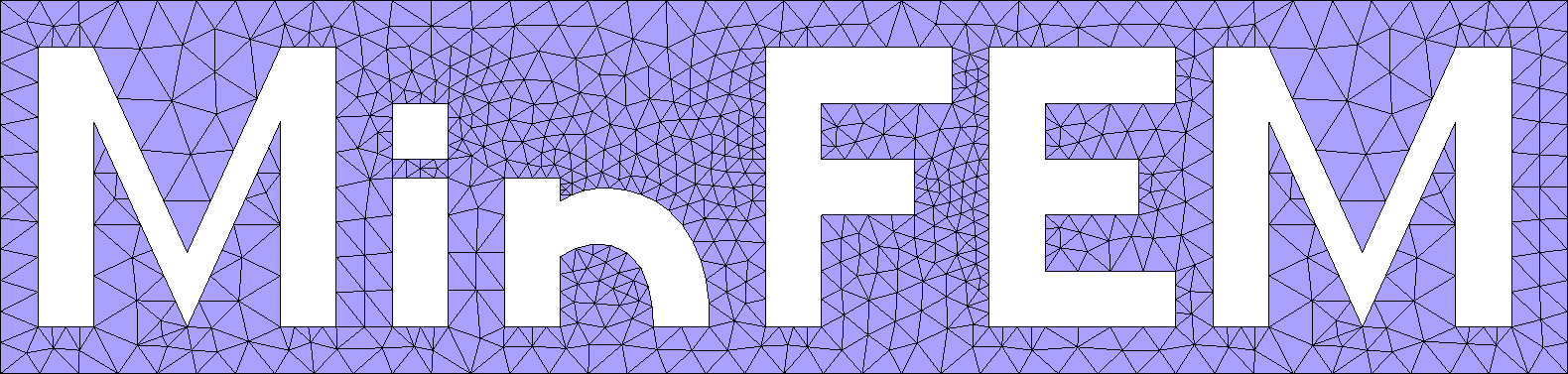## MinFEM.jl

A minimal finite element tool for demonstration and teaching.
Author msiebenborn
Popularity
1 Star
Updated Last
2 Years Ago
Started In
October 2018## A minimal finite element tool for demonstration and teaching.

• The purpose of this package is to provide an easy and minimalistic introdcution to the finite element method.

• We restrict ourselves to linear finite elements on two-dimensional, triangular grids.

• This code imports meshes in GMSH v2 and v4 format and outputs VTK format for Paraview.

First we need to add the MinFEM package to our Julia installation. Thus, hit the ] key and type

``````add MinFEM
test MinFEM
``````

Lets go through a code for the Poisson equation on a unit square with homogeneous Dirichlet boundary conditions.

First we have to load the package MinFEM and WriteVTK. The latter is used to write the results in a format suitable for Paraview. We then generate a uniform, triangular 30x30 mesh for the unit square.

```using MinFEM

mesh = unit_square(30)```

As an alternative: Download the package from github to obtain the examples and meshes and navigate, within the julia console, to the examples folder. Then import one of the mesh files generated with GMSH.

`mesh = import_mesh("../meshes/poisson.msh")`

The next step is to assemble the matrices which discretize the weak formulation:

```L = asmLaplacian(mesh)
M = asmMassMatrix(mesh)```

We now want to set s as an eigenfunction of the Laplacian multiplied with the corresponding eigenvalue:

```n=3
m=2
f(x) = ((n*pi)^2 + (m*pi)^2) *sin(n*x*pi)*sin(m*x*pi)
s = evaluateMeshFunction(mesh, f)```

The next step is to set up a PDESystem structure, which holds all necessary information for the PDE. These are the stiffness matrix, the load vector, Dirichlet values and indices of the boundary nodes:

```boundary = union(mesh.Boundaries.Nodes,
mesh.Boundaries.Nodes,
mesh.Boundaries.Nodes,
mesh.Boundaries.Nodes)

pde = PDESystem(A=L, b=M*s, bc=zeros(mesh.nnodes), DI=boundary)```

Note that the mesh is designed to have four physical boundaries identified by the indices 1001-1004.

Finally, we solve the PDE and write the solution in a file for visualization with Paraview:

```solve(pde)
vtkfile = open_vtk_file(mesh, "poisson.vtu")
write_point_data(vtkfile, pde.state, "Y")
write_point_data(vtkfile, s, "S")
save_vtk_file(vtkfile)```

### Used By Packages

No packages found.